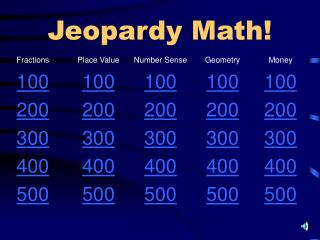# Jeopardy Math! - PowerPoint PPT PresentationDownload PresentationJeopardy Math!

Jeopardy Math!Download Presentation## Jeopardy Math!

- - - - - - - - - - - - - - - - - - - - - - - - - - - E N D - - - - - - - - - - - - - - - - - - - - - - - - - - -
##### Presentation Transcript

1. Jeopardy Math! Fractions Place Value Number Sense Geometry Money 100100100100100 200200200200200 300300300300300 400400400400400 500500500500500

2. What fraction of the rectangle is shaded? 2/4 = 1/2 = one half Fractions for 100

3. Mr. K. has 3 pencils. Two of them are red. What fraction are red? 2/3 two thirds Fractions for 200

4. Which would be the biggest piece of a pizza? 1/3 , 1/2 , 1/4 1/2 one half Fractions for 300

5. What is 1/2 of 14? 1/2 of 14 = 7 Fractions for 400

6. Imari has 2 books, LaTerra has 1 book, and Quran has 3 books. What fraction of the books does LaTerra have? 1/6 one sixth Fractions for 500

7. Which digit in the number 473 is in the ten’s place? 7 seven Place Value for 100

8. Which digit in the number 4328 is in the hundred’s place? 3 three Place Value for 200

9. What is the value of the 6 in the number 761? 60 sixty Place Value for 300

10. I have a 0 in my ten’s place, a 2 in my hundred’s place, and a 5 in my one’s place. What number am I? 205 Two hundred five Place Value for 400

11. Which digit is in the thousand’s place in the number 75842? 5 five Place Value for 500

12. Which number comes before 25? 24 twenty four Number Sense for 100

13. What is the 3rd number after 38? 41 forty one Number Sense for 200

14. Which number could come between 31 and 49? 28 42 15 42 forty two Number Sense for 300

15. What number does the arrow show on the number line? 37 . . . . . . 44 . . . . . 42 forty two Number Sense for 400

16. 100 + 70 + 6 = 176 one hundred seventy-six Number Sense for 500

17. What shape is shown below? triangle Geometry for 100

18. Which figure does not show a line of symmetry? the circle Geometry for 200

19. What shape is shown below? cylinder Geometry for 300

20. How many sides does the figure below have? 8 eight Geometry for 400

21. Which shows parallel lines? b Geometry for 500 b c a

22. Five pennies are the same as a: quarter dime nickel dollar nickel or 5 cents or 5¢ or \$.05 Money for 100

23. Two nickels are the same as a: quarter dime penny dollar dime or 10 cents or 10¢ or \$.10 Money for 200

24. I have a quarter, a dime, a nickel, and a penny. How much money do I have? forty one centsor41 centsor41¢or \$.41 Money for 300

25. I have 20 cents. If I buy a pencil for 10 cents, how much money do I have left? a dime or 10 cents or 10¢ or \$.10 Money for 400

26. I have 3 coins. Which amount could I have? 8¢ 22¢ 16¢ 32¢ 16¢ (dime, nickel, penny) Money for 500